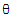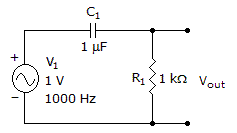# Electronics - Capacitors

### Exercise :: Capacitors - True or False

21.

A capacitor stores electric energy in the form of an electric field.

 A. True B. False

Explanation:

No answer description available for this question. Let us discuss.

22.

The frequency response curve for a low-pass filter illustrates that as the frequency of the input increases, the voltage of the output will decrease.

 A. True B. False

Explanation:

No answer description available for this question. Let us discuss.

23.

The phase angleof an RC series circuit is= invtan (XC/R) = invtan (VC/VR).

 A. True B. False

Explanation:

No answer description available for this question. Let us discuss.

24.

The true power in an RC series circuit is PR = I2 x R.

 A. True B. False

Explanation:

No answer description available for this question. Let us discuss.

25.

This circuit is a low-pass filter.A. True B. False